[file:///W:/top4.html]
 MULTIPLE CHOICE TEST RUNGE-KUTTA 2nd ORDER METHOD ORDINARY DIFFERENTIAL EQUATIONS Pick the most appropriate answer.
 Q1. To solve the ordinary differential equation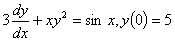by the Runge-Kutta 2nd order method, you need to rewrite the equation as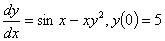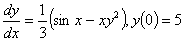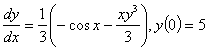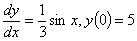Q2. Given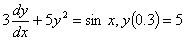and using a step size of h=0.3, the value of y(0.9) using the Runge-Kutta 2nd order Heun's method is most nearly -4297.4 -4936.7 -0.21336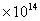-0.24489Q3. Given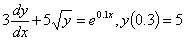,   and using a step size of h=0.3, the best estimate of dy/dx(0.9) using the Runge-Kutta 2nd order midpoint-method most nearly is -2.2473 -2.2543 -2.6188 -3.2045 Q4. The velocity (m/s) of a body is given as a function of time (seconds) by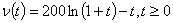Using the Runge-Kutta 2nd order Ralston method with a step size of 5 seconds, the distance in meters traveled by the body from t=2 to t=12 seconds is estimated most nearly is 3904.9 3939.7 6556.3 39397 Q5. The Runge-Kutta 2nd order method can be derived by using the first three terms of the Taylor series of writing the value of yi+1 (that is the value of y at xi+1 ) in terms of yi  (that is the value of y at xi) and all the derivatives of y at xi .  If h=xi+1-xi, the explicit expression for yi+1 if the first three terms of the Taylor series are chosen for solving the ordinary differential equation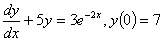would be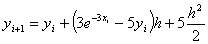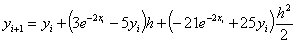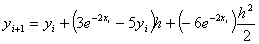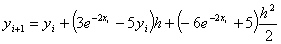Q6. A spherical ball is taken out of a furnace at 1200K and is allowed to cool in air. Given the following, radius of  ball = 2 cm specific heat of ball = 420 J/(kg-K) density of ball = 7800 convection coefficient = 350 J/s-m^2-K The ordinary differential equation is given for the temperature,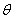of the ball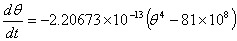if only radiation is accounted for.  The ordinary differential equation if convection is accounted for in addition to radiation is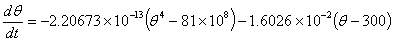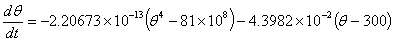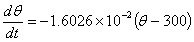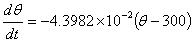[file:///W:/bottom4.html]